• 解矩阵方程+矩阵乘法，已封装，可直接调用， // private static double[][] matrixC ; /** * * 解矩阵方程 * * @param A * 矩阵 * @param b * 列阵 * @return matrixC 列阵 */ /** * * 矩阵相乘 * ...
• 用Python解矩阵方程，可以用两个模块——Numpy和Sympy 矩阵方程：Ax=b A为系数矩阵，b为解集矩阵 令B为A的增广矩阵 1、Ax=b无解的充要条件：r(A)+1=r(B) 2、Ax=b唯一解的充要条件：r(A)=r(B)=n 3、Ax=b无穷多解的充...
用Python解矩阵方程，可以用两个模块——Numpy和Sympy 矩阵方程：Ax=b A为系数矩阵，b为解集矩阵 令B为A的增广矩阵 1、Ax=b无解的充要条件：r(A)+1=r(B) 2、Ax=b唯一解的充要条件：r(A)=r(B)=n 3、Ax=b无穷多解的充要条件：r(A)=r(B)＜n
1、Numpy
用Python解矩阵方程——Numpy模块
2、Sympy
求解的逻辑： x=A-1b
from sympy import *

p1 = Matrix([[1,2],[3,4]])
y = Matrix([,])
print(p1**(-1)*y)
p2 = Matrix([[1,3,4],[2,3,4]])
print(p1**(-1)*p2)
p3 = Matrix([[1,0,3],[2,5,4],[3,4,5]])
p4 = Matrix([[1,3,4],[2,7,4],[6,5,8]])
print(p3**(-1)*p4)

结果：
Matrix([[-1], ])
Matrix([[0, -3, -4], [1/2, 3, 4]])
Matrix([[19/4, -3, 3], [-1/2, 1, -2/3], [-5/4, 2, 1/3]])

如果A不是可逆矩阵，那么矩阵方程无法进行求解 将会报错：
sympy.matrices.common.NonSquareMatrixError

展开全文python 线性代数 Sympy
• 用Python解矩阵方程，可以用两个模块——Numpy和Sympy 矩阵方程：Ax=b A为系数矩阵，b为解集矩阵 令B为A的增广矩阵 1、Ax=b无解的充要条件：r(A)+1=r(B) 2、Ax=b唯一解的充要条件：r(A)=r(B)=n 3、Ax=b无穷多解的充...
用Python解矩阵方程，可以用两个模块——Numpy和Sympy 矩阵方程：Ax=b A为系数矩阵，b为解集矩阵 令B为A的增广矩阵 1、Ax=b无解的充要条件：r(A)+1=r(B) 2、Ax=b唯一解的充要条件：r(A)=r(B)=n 3、Ax=b无穷多解的充要条件：r(A)=r(B)＜n
1、Numpy
这里要用到numpy.linalg模块
import numpy as np
# numpy.linalg模块包含线性代数的函数。使用这个模块，可以计算逆矩阵、求特征值、解线性方程组以及求解行列式等
from numpy.linalg import *

p1 = np.array([[1,2],[3,4]])
y = np.array([,])
print(solve(p1,y))
p2 = np.array([[1,3,4],[2,3,4]])
print(solve(p1,p2))
p3 = np.array([[1,0,3],[2,5,4],[3,4,5]])
p4 = np.array([[1,3,4],[2,7,4],[6,5,8]])
print(solve(p3,p4))

结果：
[[-1.]
[ 3.]]
[[ 0.  -3.  -4. ]
[ 0.5  3.   4. ]]
[[ 4.75       -3.          3.        ]
[-0.5         1.         -0.66666667]
[-1.25        2.          0.33333333]]

用这个模块求解矩阵方程时，有一个缺点，就是： 系数矩阵A必须为n×n 相应的b矩阵为n×m 如果矩阵A不满足n×n，会出现下面的情况：
import numpy as np
# numpy.linalg模块包含线性代数的函数。使用这个模块，可以计算逆矩阵、求特征值、解线性方程组以及求解行列式等
from numpy.linalg import *

y = np.array([,])
p2 = np.array([[1,3,4],[2,3,5]])
print(solve(p2,y))

结果：
numpy.linalg.LinAlgError: Last 2 dimensions of the array must be square

2、Sympy
用Python解矩阵方程——Sympy模块
展开全文python numpy 线性代数
• 在阻抗矩阵填充之后，接下来就是解矩阵方程了。后期在补上公式，先上代码。 注意：方程求得解是函数的解。 #pragma once #include<iostream> #include<stdio.h> #include<armadillo> #include&...
在阻抗矩阵填充之后，接下来就是解矩阵方程了。后期在补上公式，先上代码。
注意：方程求得解是  函数的系数。
#pragma once
#include<iostream>
#include<stdio.h>
#include<math.h>
#include<complex>
#include<time.h>
#include<cmath>
#include"FillZmat.h"

using namespace arma;
using namespace std;

extern double k;
extern mat basis_list;
extern cube element_list;
extern int elem_num;
extern int rwg_num;
extern mat Center;
extern vec Area;

extern mat CommonEdgeP;
extern mat CommonEdgeN;
extern vec EdgeLength;  //公共边长度
extern vec Vertex_pos;  // 正三角形的 非公共边点 编号
extern vec Vertex_naga;

extern cube Center_;   //九点积分划分后的小三角形重心

extern mat Pho_Pos;       //对于正element三角形 非公共边点到重心的向量坐标
extern mat Pho_Naga;      //对于负element三角形 非公共边点到重心的向量坐标
extern cube Pho_Pos_;     //对于正element三角形 九点划分后 非公共边点到 小三角形重心的向量坐标
extern cube Pho_Naga_;    //对于负element三角形 九点划分后 非公共边点到 小三角形重心的向量坐标

extern cx_mat Z;
extern rowvec Pol, dz;        //定义入射波的极化方向和入射方向(only for r_s = 1)
extern double FeedVoltage;    //定义馈电电压
extern rowvec FeedEdge;       //定义馈电边的EdgeElement编号

rowvec kv(1, 3);
cx_colvec I, V;

void SolveMat() {
kv = k * dz;
FillmatZ();
double ScalarTemp = 0.0;
cx_rowvec Einc_Pos(1, 3), Einc_Nega(1, 3);
cx_colvec Vv(rwg_num, 1), Ii(rwg_num, 1);
complex<double>EPho_Pos(0, 0), EPho_Nega(0, 0);

for (int i = 0; i < rwg_num; i++) {
ScalarTemp = as_scalar(kv * strans(Center.row(basis_list(i, 0))));
Einc_Pos = Pol * exp(complex<double>(0, -ScalarTemp));

ScalarTemp = as_scalar(kv * strans(Center.row(basis_list(i, 1))));
Einc_Nega = Pol * exp(complex<double>(0, -ScalarTemp));

EPho_Pos = as_scalar(Einc_Pos * strans(Pho_Pos.row(i)));
EPho_Nega = as_scalar(Einc_Nega * strans(Pho_Naga.row(i)));
Vv = EdgeLength(i) * (EPho_Pos / 2.0 + EPho_Nega / 2.0);
}
printf("\n 正在求解矩阵方程 ... \n");
clock_t start = clock();
Ii = solve(Z, Vv);          //注意球的的解是 RWG函数 的系数
clock_t end = clock();
cout << endl << "解方程的时间为：" << (end - start) << "ms" << endl;
V = Vv;
I = Ii;
}

展开全文• 列主元法解矩阵的值，亲测通过该代码，可以求出矩阵值。
• ## matlab解矩阵方程组

千次阅读 2020-07-11 21:47:03
解方程组 对于一个初学者来说，刚上来是这样做的 然后不出意外报错了 后来根据课件想到了matlab中求逆矩阵的函数 inv（A）； 瞬间豁然开朗 所以说还是蛮简单的 做个笔记： ps：（如果线代考试会用matlab，岂...
题意如下： 解方程组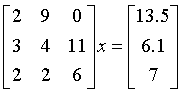对于一个初学者来说，刚上来是这样做的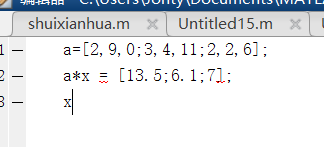然后不出意外报错了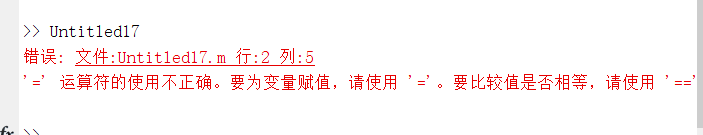后来根据课件想到了matlab中求逆矩阵的函数 inv（A）； 瞬间豁然开朗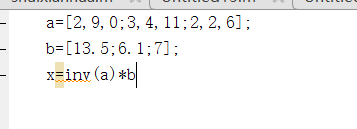所以说还是蛮简单的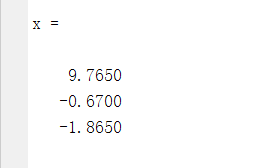做个笔记：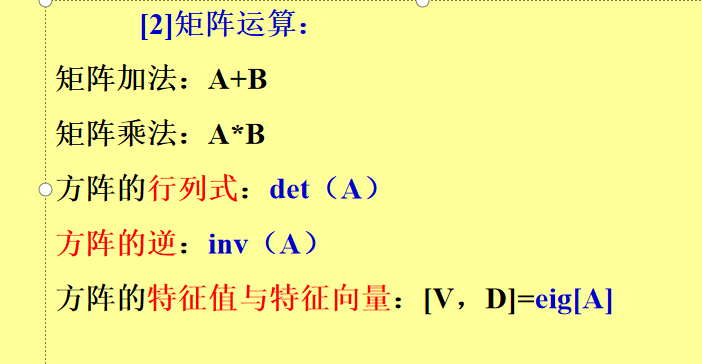ps：（如果线代考试会用matlab，岂不是美滋滋哈哈哈，特征值特征向量一分钟搞定（特指线上考试））哈哈哈哈哈嗝 拜拜~
展开全文线性代数 matlab
• 求逆 A-1 * (A|E) = (E|A-1) 对（A|E）进行初等行变换即可得（E|A-1） 解矩阵方程 A * X = B X=A-1 * B A-1 * (A|B) = (E|X) 对 (A|B) 进行初等行变换即可得（E|X）
• python numpy 解矩阵方程错误（LinAlgError Last 2 dimensions of the array must be square） 原因 由于numpy直接使用x = numpy.linalg.solve(A, B) 必须保证A是一个方阵，但是我的矩阵不是方阵 解决 凑0合成方阵...numpy python
• 苏教版小学数学五年级下册解方程式练习(260题).doc文档
• （苏）版小学数学五年级下册解方程式练习（260题）.doc文档
• 我们暂且将 矩阵看为多元方程组，（将新知识与已有知识建立连结是记忆的好方法）。 X +2y +4z 7 3x +7y +2z -11 ...线性代数
• 矩形面积是120,对角线长是17,如何获取矩形长和宽。。。。。。。。c#
• 看到了这文我震惊了，正则能二元方程式~~，需要的朋友可以看下
• 列主元法解方程组并可以替换无解矩阵，并给出有解矩阵
• ## 矩阵解方程组

千次阅读 2020-12-29 13:01:36
矩阵解方程方程组: 3x+2y=7−6x+6y=6 3x +2y = 7 \\ -6x+6y = 6 3x+2y=7−6x+6y=6 用矩阵表示: [32−66]∗[xy]= \begin{bmatrix} 3 & 2 \\ -6 & 6 \\ \end{bmatrix} * \begin{bmatrix} x \\ y \\ \...
• ## 矩阵解线性方程

千次阅读 2020-02-24 17:58:10
二元线型方程 我们熟知的两个未知数两个方程组的，等价于两条直线的交点 可能交于一点（一个），平行（无）...答案很明显了，解方程也就是对矩阵的变换 我们来看组无的情况： 当化成第一个三角形时 0=52...线性代数 机器学习 算法
• 针对摄动参数为带有范数有界不确定性的摄动离散Riccati矩阵方程解的特征值的估计问题,利用矩阵不等式和特征值等性质,得到了摄动离散Riccati矩阵方程解的特征值新的上界,这种表示只利用了特征值及奇异值计算,避免了...
• 主要介绍了基于python线性矩阵方程(numpy中的matrix类),文中通过示例代码介绍的非常详细，对大家的学习或者工作具有一定的参考学习价值,需要的朋友可以参考下
• 与研究m次代数方程相类似地研究了m次非线性矩阵方程，给出了一般解的求法，一般...这种判定方法又直接导出了m次可解矩阵方程根的公式，及非奇异矩阵的m次根的表达式．最后我们也在各种不同的情况给出了结论的数值例子．
• 有一个矩阵方程xA=B,其中x是一个1∗n的行向量,A是一个n∗n的矩阵,B是一个1∗n的行向量,并且n=9999999999999999999. 并且A[i][j]=1当且仅当i是j的约数,否则A[i][j]是0,B[i]=1当且仅当i=1,否则B[i]是0. 给出一个d和...x 数据 测试
• 楚列斯基分解 希尔伯特矩阵 解方程组 数值计算 代码 楚列斯基分解 希尔伯特矩阵 解方程组 数值计算 代码
•研究论文
• 几类四元数矩阵方程的最小二乘，张丽娜，徐东坡，四元数矩阵方程在生物学、电学、振动理论、结构设计、自动控制理论和最优控制等领域有着重要的应用。四元数矩阵方程的求解是数值
• 上回书我们讲述了线性方程组的直接求解方法，这些方法利用...概述把 改写成 ，如果这一迭代格式收敛，对这个子不断迭代计算就可以得到方程组的。道理很简单：对 两边取极限，显然如果收敛，则最终得到的满足 ...
• LU矩阵分解代码，直接输入系数矩阵，输出，A=LU，LUx=bpython
• 基于标准相关分解的非线性矩阵方程，田时瑞，邓远北，针对非线性二次矩阵方程AX2+BX=C,应用矩阵对的标准相关分解(CCD),导出了问题的的存在条件,并给出了方程的极小范数以及方程的对称�
• 利用行列计算线性方程矩阵初等变换法线性方程等代码。其中初等变换法计算行列线性方程不限价数，可无限多价齐次方程。递归法因为损耗内存具大，只适用于30*30以下的行列或线性方程，但对编程初学...
• 矩阵的基本运算;矩阵的基本运算;矩阵的特征值特征向量特征多项式; 线 性 方 程 组;...例5-21 求非齐次线性方程组的通 MATLAB命令为 B=[1 -1 -1 1 0;1 -1 1 -3 1;1 -1 -2 3 -1/2]; rref(B) ans = 1
• ## python解多元方程式

千次阅读 2019-09-26 14:40:39
例 x = Symbol('x') y = Symbol('y') z = Symbol('z') r = solve([ (x + y) / 2 - (y + z) / 3, (x + y) / 2 - (x + z) / 4, (y + z) / 3 - (x + z) / 4, x + 2 * y + z - 12 ], [x, y, z......